czt
pypi i czt

pypi i czt

# Chirp Z-Transform (CZT)From Wikipedia:

The chirp Z-transform (CZT) is a generalization of the discrete Fourier transform (DFT). While the DFT samples the Z plane at uniformly-spaced points along the unit circle, the chirp Z-transform samples along spiral arcs in the Z-plane, corresponding to straight lines in the S plane. The DFT, real DFT, and zoom DFT can be calculated as special cases of the CZT.

## Getting Started

You can install the CZT package using `pip`:

``````# to install the latest release (from PyPI)
pip install czt

git clone https://github.com/garrettj403/CZT.git
cd CZT
pip install -e .

# to install dependencies for examples
pip install -e .[examples]
``````

## Example

Consider the following time-domain signal: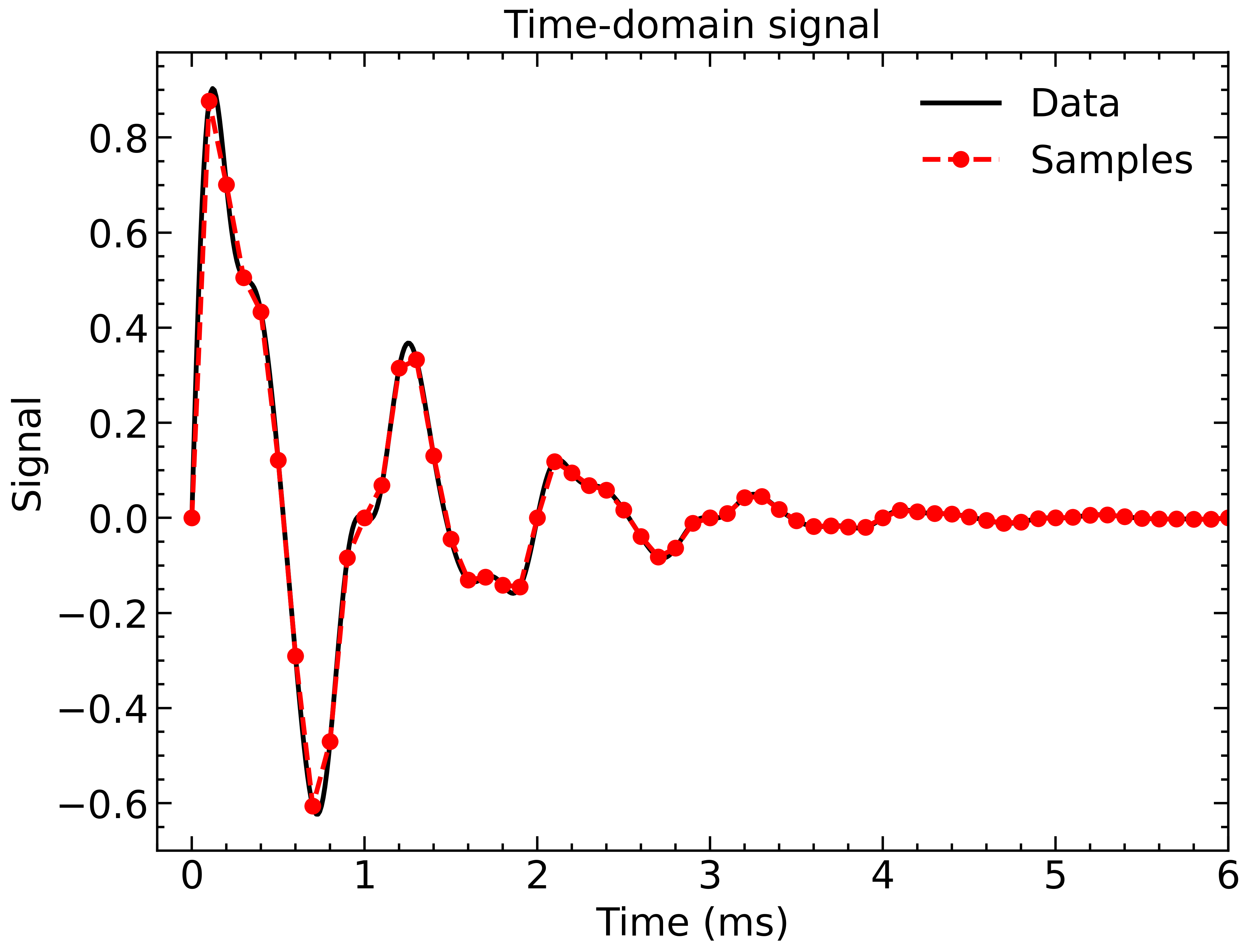This is an exponentially decaying sine wave with some distortion from higher-order frequencies. We can convert this signal to the frequency-domain to investigate the frequency content using the Chirp Z-Transform (CZT):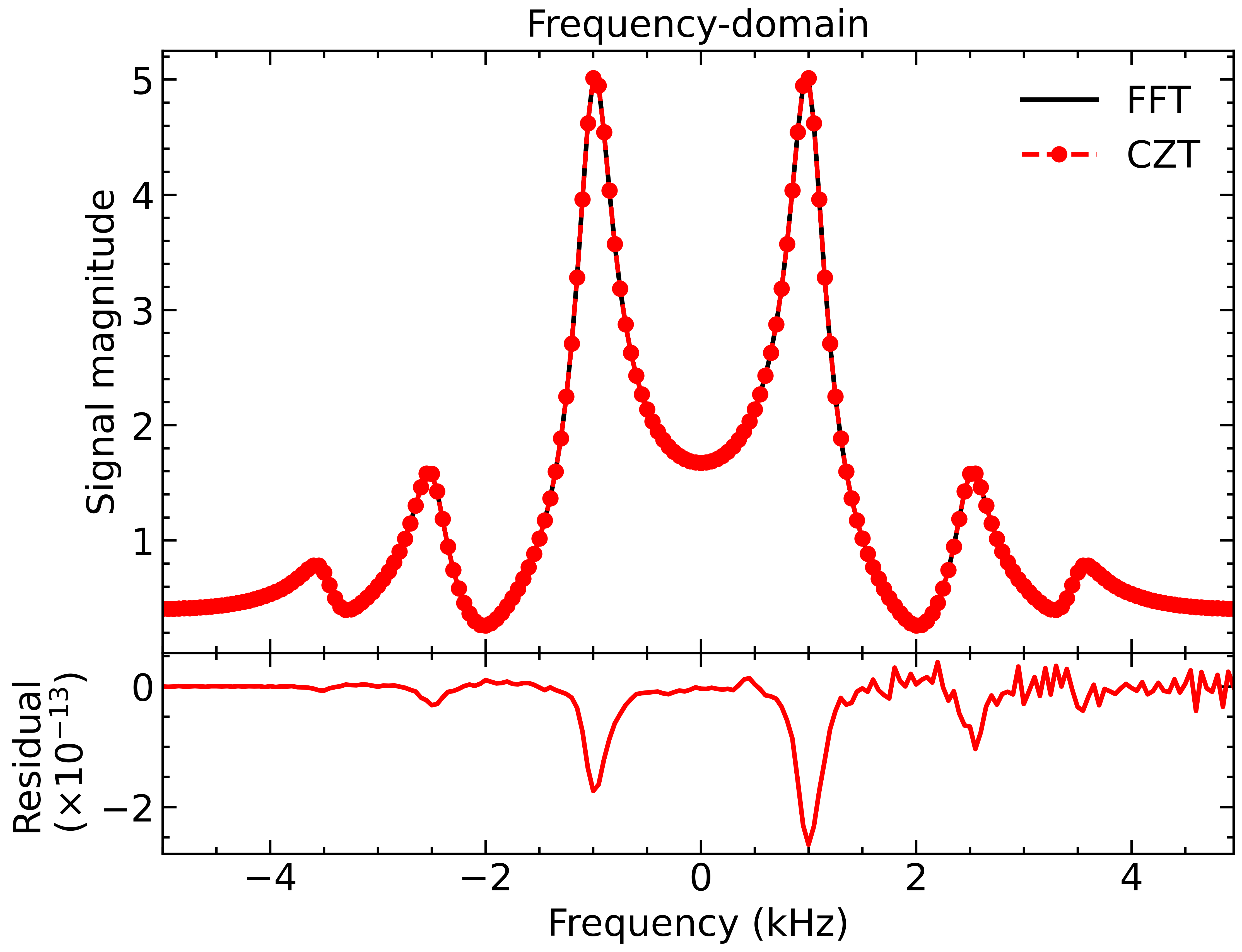Note that the CZT also allows us to calculate the frequency response over an arbitrary frequency range: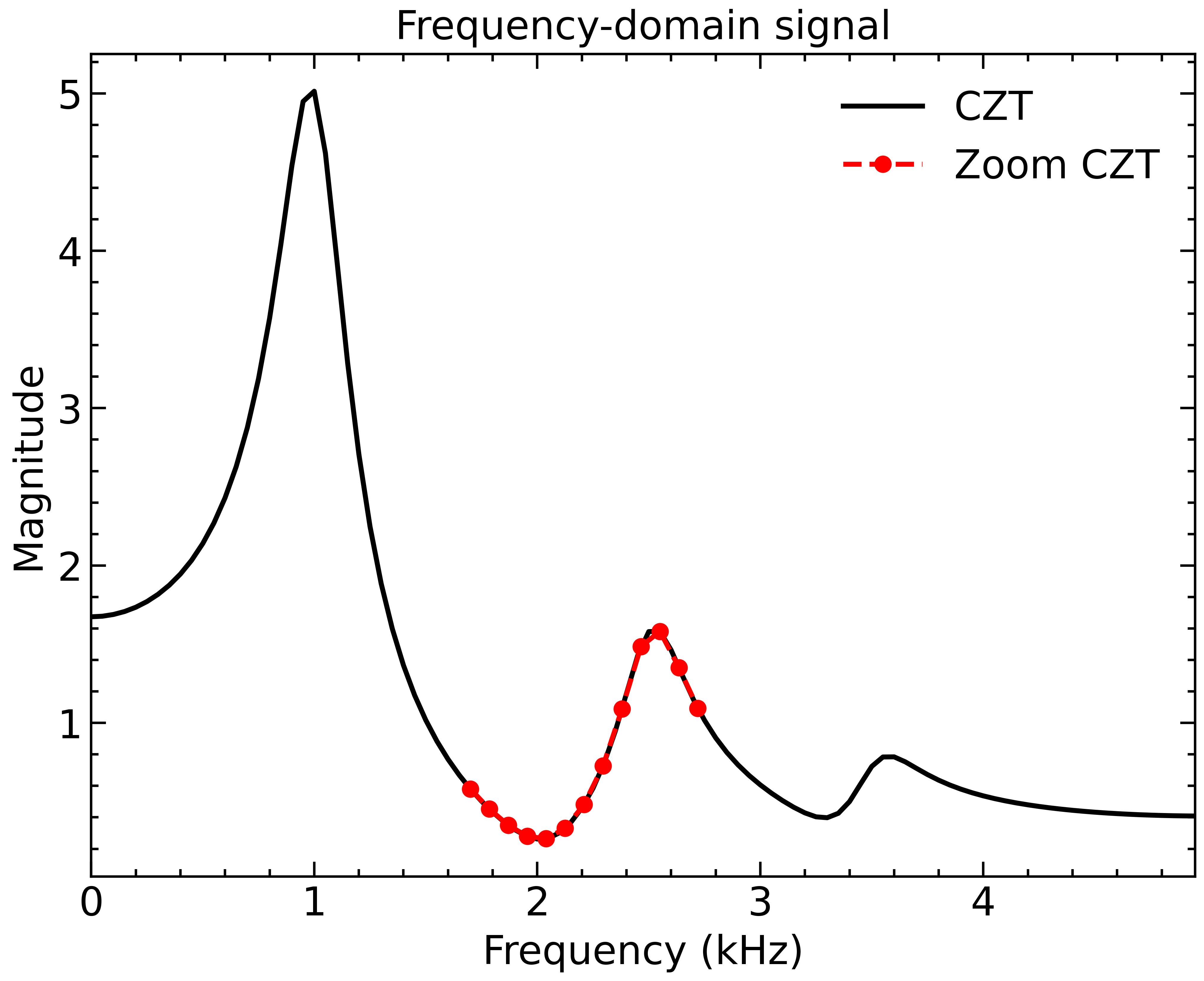We can see that the signal has frequency components at 1 kHz, 2.5 kHz and 3.5 kHz. To remove the distortion and isolate the 1 kHz signal, we can apply a simple window in the frequency-domain: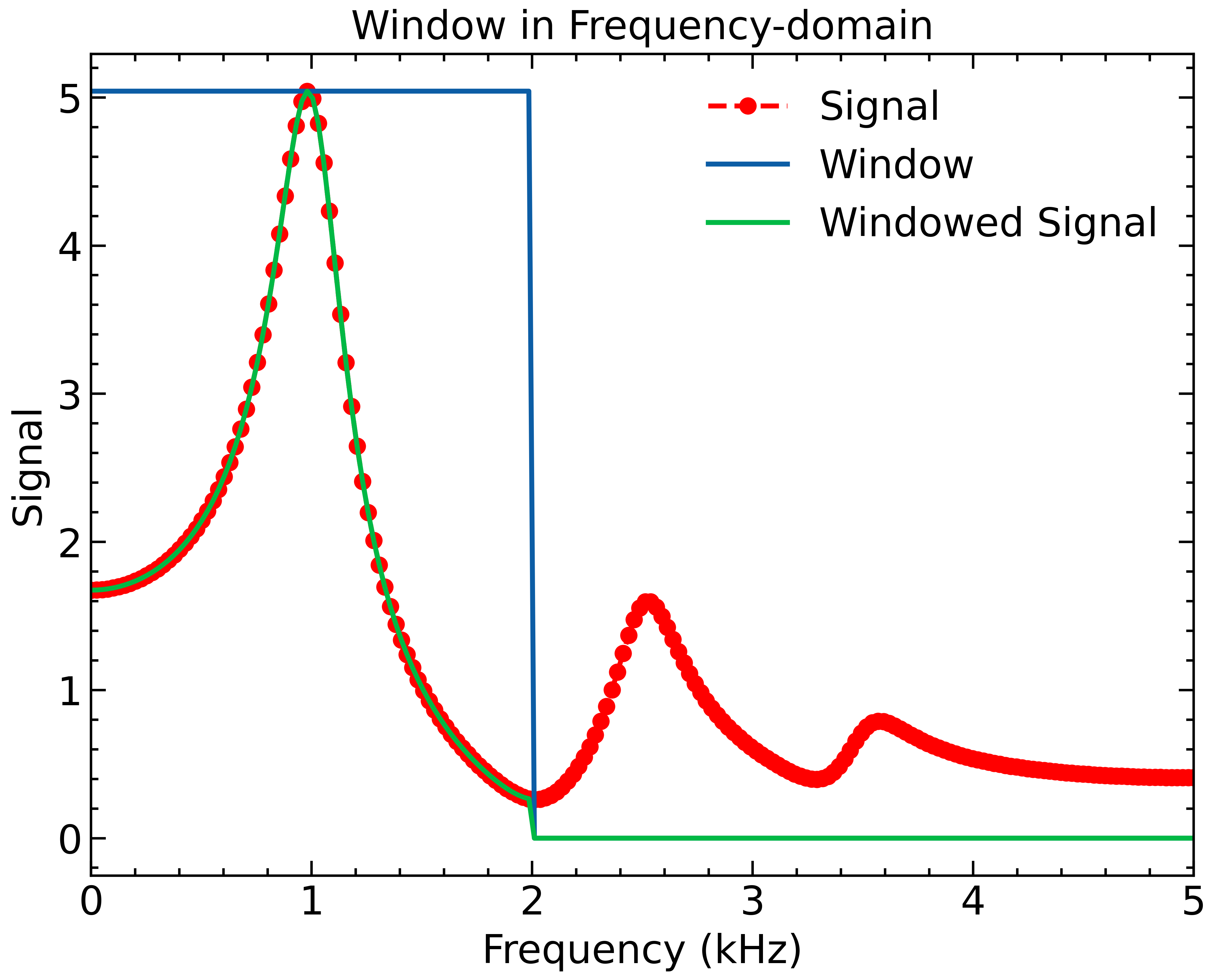Finally, we can use the Inverse Chirp Z-Transform (ICZT) to transform back to the time domain: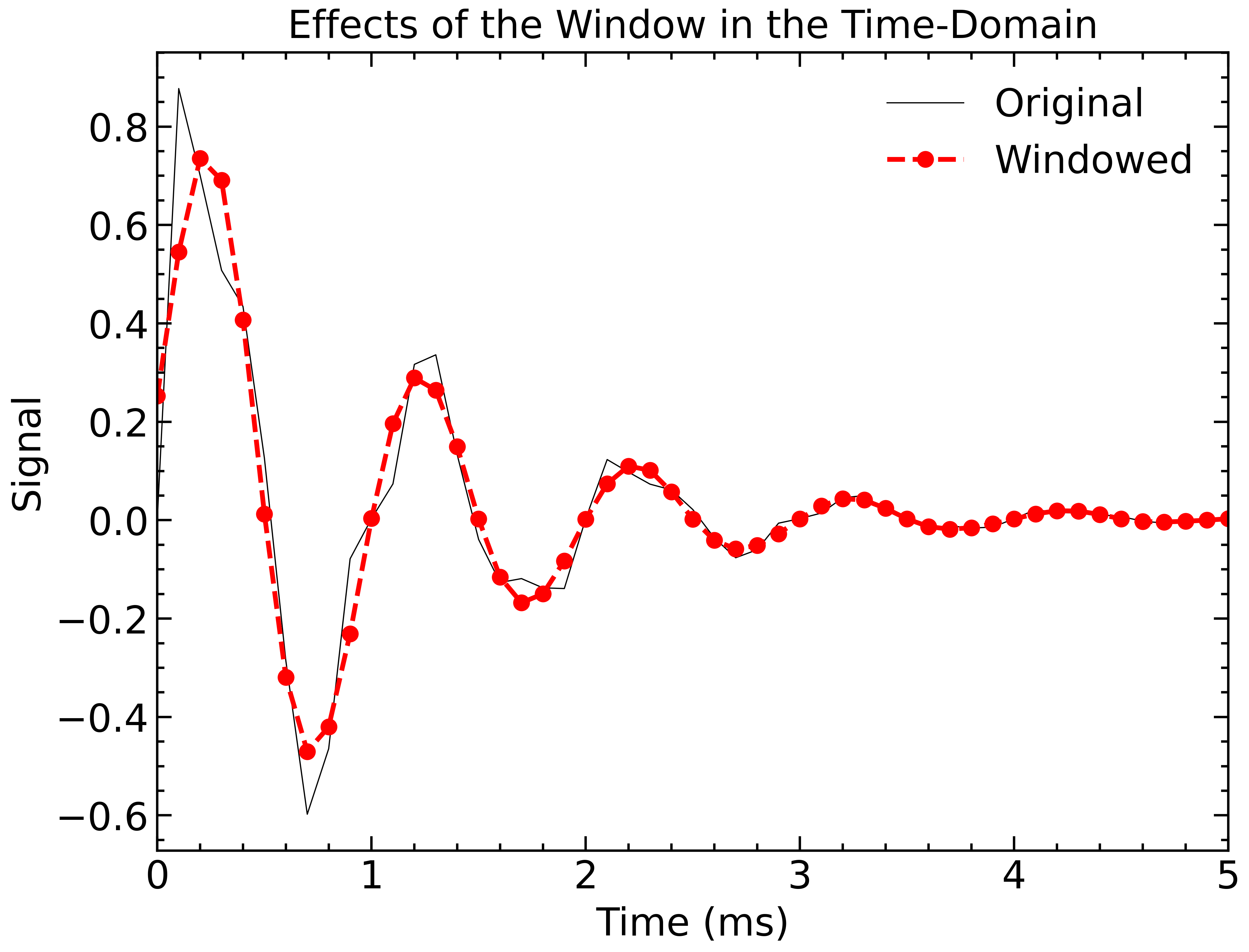As we can see, we were able to remove the higher-order frequencies that were distorting our 1 kHz signal.

You can find this example and others in the `examples/` directory.

13

10mos ago

1

4

0

### OPEN PRs

0
VersionTagPublished
0.0.7
10mos ago
0.0.6
1yr ago
0.0.5
1yr ago
0.0.4
1yr ago